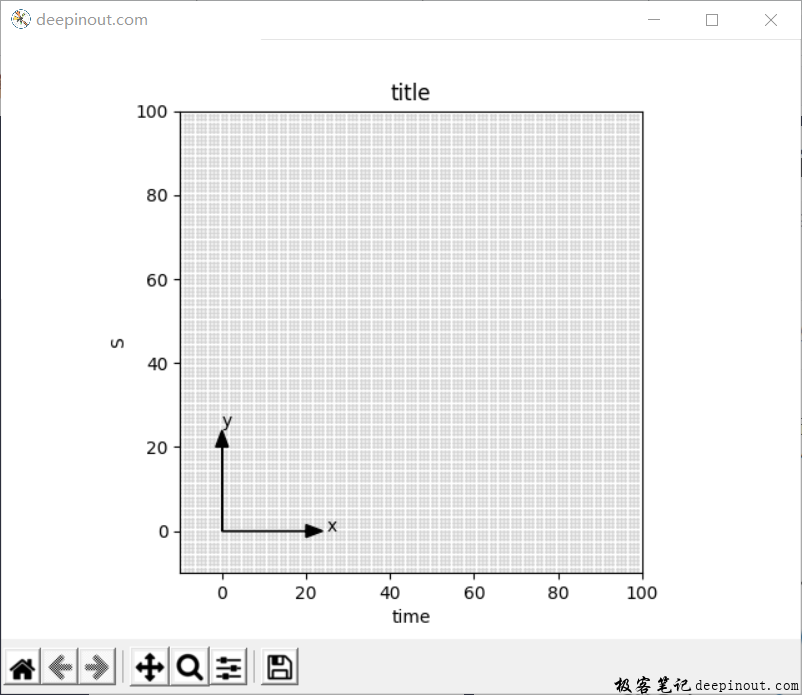# Matplotlib 辅助指示坐标轴``````import matplotlib.pyplot as plt
import numpy as np

x1, x2 = -10, 100
y1, y2 = -10, 100

fig = plt.figure('deepinout.com')
plt.axes().set_aspect('equal')
plt.axis([x1, x2, y1, y2]) #

plt.xlabel('time')
plt.ylabel('S')
plt.title('title')

dx = 1
dy = 1
for x in np.arange(x1, x2, dx):
for y in np.arange(y1, y2, dy):
plt.scatter(x, y, s = 1, color = 'lightgray')

#画一个箭头坐标系指示
plt.arrow(0, 0, 20, 0, head_length = 4, head_width = 3, color = 'k')
plt.arrow(0, 0, 0, 20, head_length = 4, head_width = 3, color = 'k')
plt.text(25, 0, 'x')
plt.text(0, 25, 'y')

plt.show()
``````

• 微信订阅
• 回顶